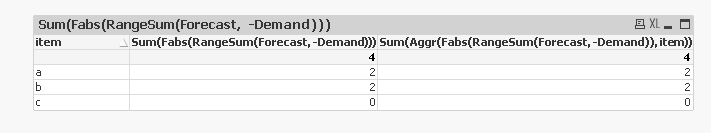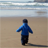# QlikView App Dev

Discussion Board for collaboration related to QlikView App Development.

Announcements
On May 18th at 10AM EDT we will answer your QlikView questions live. REGISTER
cancel
Showing results for
Did you mean:Not applicable

## In Pivot table, How to fabs firstly then sum

Hi, Guys

I met a big problem on ABS function

I need to get the final result is sum all the item absloute deviation

for example

itemForecastDemand
a1012
b108
c1010

The correct anwser is |10-12|+|10-8|+|10-10| = 4

not |10+10+10 - 12-8-10| = 0

From my previous experience, I create a temp table to store each absloute deviation, the put them in the pivot table

However, the new case is the Demand is composed of three kinds of Demand

Take first row 12 as example, 12 comes from  8(current)+4 (future) + 0 (old)

The user want to add a filter to customize the real Demand (8+4 or 8 lonely)

Then how do I create the  temp table to calculate each independent absloute deviation to suit for the filter?

1 Solution

Accepted SolutionsMVP

May be something like this;

Sum(Fabs(RangeSum(Forecast, Demand))) or

Sum(Aggr(Fabs(RangeSum(Forecast, Demand)), Item))

Sum(Fabs(RangeSum(Forecast, -Demand))) or

Sum(Aggr(Fabs(RangeSum(Forecast, -Demand)), Item))13 RepliesChampion

if Forecast and Demand are two expression in your chart, then use 3rd expression like:

Forecast - Demand  //Expression label name, you can use abs() as wellMVP

May be something like this;

Sum(Fabs(RangeSum(Forecast, Demand))) or

Sum(Aggr(Fabs(RangeSum(Forecast, Demand)), Item))

Sum(Fabs(RangeSum(Forecast, -Demand))) or

Sum(Aggr(Fabs(RangeSum(Forecast, -Demand)), Item))Not applicable
Author

in item level it worked

but if in higher level, the summary will sum first then absPartner

Hi,

You can use this as your expression

sum(aggr(fabs(Forecast-Demand),Item))

Hope this help

Regards,

MarcoMVP

Hi,

You can try using Aggr() like below

=Sum(Aggr(Fabs(Forecast-Demand), item))

OR the best thing is calculate in the script itself

*,

Forecast - Demand AS ForecastDiff

FROM DataSource;

Now you can straight away use

=Sum(ForecastDiff)

Note: If data is huge Aggr() will have performance issues.

Hope this helps you.

Regards,

Jagan.MVP

Hi,

did you try simple expression like

sum(fabs(Forecast-Demand))

This will work.

Regards

Great dreamer's dreams never fulfilled, they are always transcended.Not applicable
Author

Thanks for your guys anwser, the total amount will be aggrated on the top by the straight table

But in addition, if i want to display in month level ,for example

201505     2

201506     4

In pivot table it may expand to monthly item level, but here we knows must use straight table , so how to display the month level abs?

I need a chart to describe the monthly trend, so i need each month point abs first then sumNot applicable
Author

Thanks for your guys anwser, the total amount will be aggrated on the top by the straight table

But in addition, if i want to display in month level ,for example

201505     2

201506     4

In pivot table it may expand to monthly item level, but here we knows must use straight table , so how to display the month level abs?

I need a chart to describe the monthly trend, so i need each month point abs first then sum chooco_coPartner

Hi Jarven,,